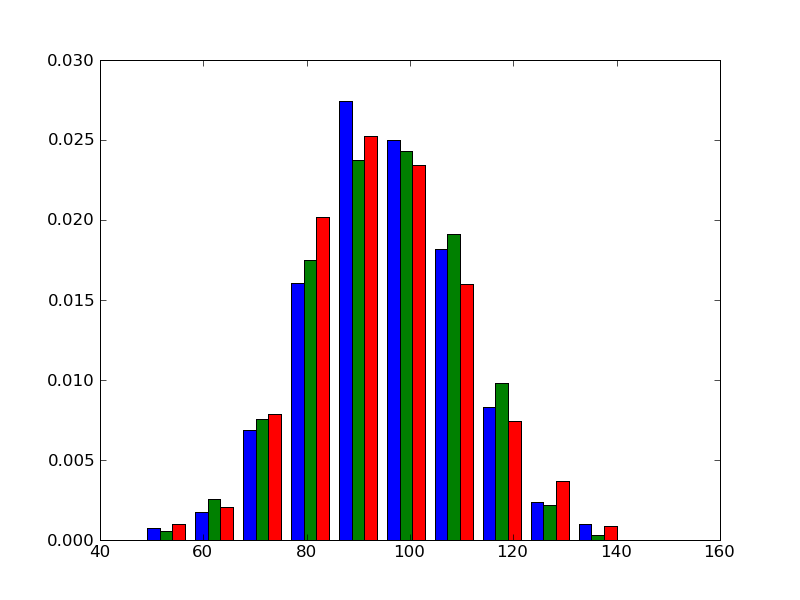# hist again

Hi,
I had one or two more looks at the hist() function. There are a few things I wondered about:

(I) Isn't it more intuitive to interpret the "width" keyword as "width relative to the real width of a bin" rather than as an absolute value ? Here is an example, why I think so: Say I want to create a histogram where the individual bars touch each other. First create some data

In : sigma * 38.
In : Y = sigma * numpy.random.randn(1000)
In : pylab.hist(Y)

By default, this produces a histogram where there is some space between the bars. But how should I now (in advance) what the width will be? That depends on the retuned bins of the numpy.histogram routine, so the only direct solution would be

In : n, bins, patches = pylab.hist(Y)
In : pylab.clf()
In : n, bins, patches = pylab.hist(Y, width=bins-bins)

(II) If width < real_width_of_a-bin, why is the bar aligned to the left edge of the bin, not to its center? (That different from the align='center' behaviour). Try a width that is << real_width_of_a-bin . The result looks strange to me and is hard to interpret.

(III) Now the real interesting thing !!! matlab has the ability to create a kind of combined histogram, if the input is not an 1d array, but a matrix. So, I played a little bit around and added such a feature to the matplotlib hist method. It isn't finished yet, but might be of interest ???

from pylab import *
import numpy
mu, sigma = 100, 15
x = mu + sigma*numpy.random.randn(1000,3)
ret = hist(x, 10, normed=True)
show()

... produces a figure as attached.

ManuelDear all,

as there was no disagreeing feedbackI continued my work on the hist() method. I just committed a patch with some major re-writing of the hist() method to the trunk. I personally think it is very useful. hist() now

- supports 2D input data (i.e. multiple data, but not yet list of
arrays with different length; is a TODO)
- supports "stacked" histograms for multiple data
- the 'edge' alignment has been changed to align a bar in the center
between two edges rather than on the left edge of a bin. This seems
be more convenient (to me) and plots are easier to interpret
- the width keyword is Deprecated, and the new keyword rwidth is
introduced to give the *relative width* of a bar rather than an
absolute value (i.e. rwidth = 0.8 means the width of the bar is 80%
of the width of the bin), this also works for *unequally* spaced
bins

- I added an example histogram_demo_extended.py to show how the new
features work / look -- I like itThese changes also mean some minor API breakings (alignment='edge'; width deprecated), but as hist() in the trunk has switched to future numpy.histogram(), users have to check there code anyway.

I am, however, not very happy with the align keywords. I have more or less left this as is, but don't find them very logical: 'center' means centered on the left bin-edge, and 'edge' means centered on the center of the binManuel